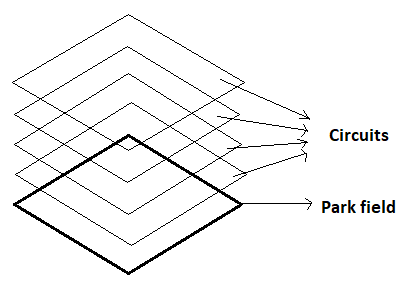QUESTION

# A square shape of park of area 23,104 sq. m is to be enclosed with wire placed at heights 1, 2, 3, 4 m above the ground. Find the required length of the wire, if its length required for each circuit is 10% greater than the perimeter of the field.

Hint: Let us find the side length of the park by equating the formula of area of square (i.e. ${a^2}$) with the given area of the park. And then find the length of wire required for each circuit using a percentage formula.As we know that the shape of the park is square.
And the formula for the area of the park is ${a^2}$, where a is the side length of the square.
So, to find the value of the side length of the park we equate that with the given area of the park.
Let the length of the side of the park is a metre.
So, ${a^2}$ = 23104
So, a = 152 metres.
As we know that the formula for the perimeter of the square is 4a, where a is the side length of the square.
So, the perimeter of the field will be 4a = 4*152 metres = 608 metres.
Now as it is given in the length required for each circuit is 10% greater than the perimeter of the field.
So, now we have to use a percentage formula which states that A% of B is calculated as $\dfrac{A}{{100}} \times B$.
So, now 10% of the perimeter will be equal to $\dfrac{{10}}{{100}} \times 608$ = 60.8 metres.
So, the length of the wire required for each circuit will be equal to the summation of the perimeter of the field and 10% of the perimeter of the field.
So, the length of wire required for one circuit will be = 608 + 60.8 = 668.8 metres.
As we know that there are four circuits 1, 2, 3 and 4 m above the level of ground.
So, total length of the wire required will be equal to 4*668.8 = 2675.2 metres
Hence, the required length of the wire will be 2675.2 metres.

Note: Whenever we come up with this type of problem then first, we have to find the side length of the field by comparing the given area with the formula of area of square. And after that we had to find the perimeter of the field by using perimeter formula and then on using the percentage formula we will find the 10% of the perimeter and after that on adding 10% of the perimeter with the perimeter with the field we will get the length of wire required for one circuit and then after multiplying that with four we will get the required length of the wire.